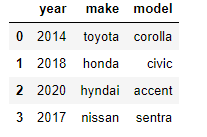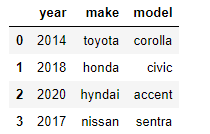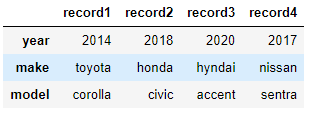# 15 Ways of Creating a DataFrame with PandasA learner’s reference for different ways of creating a DataFrame with Pandas: Using DataFrame constructor pd.DataFrame(); Using pandas library functions — read_csv, read_json; From other dataframes

## Motivation

While doing EDA (exploratory data analysis) or developing / testing models, it is very common to use the powerful yet elegant pandas DataFrame for storing and manipulating data. And usually, it starts with “creating a dataframe”.

I usually encounter the following scenarios while starting some EDA or modeling with pandas:

I need to quickly create a dataframe of a few records to test a code. I need to load a csv or json file into a dataframe. I need to read an HTML table into a dataframe from a web page I need to load json-like records into a dataframe without creating a json file I need to load csv-like records into a dataframe without creating a csv file I need to merge two dataframes, vertically or horizontally I have to transform a column of a dataframe into one-hot columns

Each of these scenarios made me google the syntax or lookup the documentation every single time, until I slowly memorized them with practice of months and years.

Understanding the pain it took to lookup, I thought a quick lookup sheet for the multiple ways to create a dataframe in pandas may save some time. This may help learners until they become seasoned data analysts or data scientists.

So here are a few ways we can create a dataframe. If anyone reading this finds other elegant ways or methods, please feel free to comment or message me; I would love to add them in this page with your reference.

## Using DataFrame constructor pd.DataFrame()

The pandas DataFrame() constructor offers many different ways to create and initialize a dataframe.

• Method 0 — Initialize Blank dataframe and keep adding records. The columns attribute is a list of strings which become columns of the dataframe. DataFrame rows are referenced by the loc method with an index (like lists). For example, the first record in dataframe df will be referenced by df.loc, second record by df.loc. A new row at position i can be directly added by setting df.loc[i] =
``````## method 0

## Initialize a blank dataframe and keep adding
df = pd.DataFrame(columns = ['year','make','model'])
## Add records to dataframe using the .loc function
df.loc = [2014,"toyota","corolla"]
df.loc = [2018,"honda","civic"]
df``````
• Method 1 — using numpy array in the DataFrame constructor. Pass a 2D numpy array — each array is the corresponding row in the dataframe
``````## Pass a 2D numpy array - each row is the corresponding row required in the dataframe

data = np.array([[2014,"toyota","corolla"],
[2018,"honda","civic"],
[2020,"hyndai","accent"],
[2017,"nissan","sentra"]])

## pass column names in the columns parameter
df = pd.DataFrame(data, columns = ['year', 'make','model'])
df``````• Method 2 — using dictionary in the DataFrame constructor. Dictionary Keys become Column names in the dataframe. Dictionary values become the values of columns. Column values are combined in a single row according to the order in which they are specified
``````data = {'year': [2014, 2018,2020,2017],
'make': ["toyota", "honda","hyndai","nissan"],
'model':["corolla", "civic","accent","sentra"]
}

## pass column names in the columns parameter
df = pd.DataFrame(data)
df``````• Method 3 — using a list of dictionaries in the DataFrame constructor. Each dictionary is a record. Dictionary Keys become Column names in the dataframe. Dictionary values become the values of columns
``````data = [{'year': 2014, 'make': "toyota", 'model':"corolla"},
{'year': 2018, 'make': "honda", 'model':"civic"},
{'year': 2020, 'make': "hyndai", 'model':"nissan"},
{'year': 2017, 'make': "nissan" ,'model':"sentra"}
]
## pass column names in the columns parameter
df = pd.DataFrame(data)
df``````• Method 4 — using dictionary in the from_dict method. Dictionary Keys become Column names in the dataframe. Dictionary values become the vaues of columns. Column values are combined in a single row according to the order in which they are specified_._
``````data = {'year': [2014, 2018,2020,2017],
'make': ["toyota", "honda","hyndai","nissan"],
'model':["corolla", "civic","accent","sentra"]
}

## pass column names in the columns parameter
df = pd.DataFrame.from_dict(data)
df``````Note: There is a difference between methods 2 and 4 even though both are dictionaries. Using from_dict, we have the ability to chose any column as an index of the dataframe. What if the column names we used above need to be indexes — like a transpose of the earlier data ? Specify orient = “index” and pass column names for the columns generated after the transpose

``````df = pd.DataFrame.from_dict(data, orient='index',columns=['record1', 'record2', 'record3', 'record4'])
df``````## Basic Data Types in Python | Python Web Development For Beginners

In the programming world, Data types play an important role. Each Variable is stored in different data types and responsible for various functions. Python had two different objects, and They are mutable and immutable objects.

## Hire Python Developers

Are you looking for experienced, reliable, and qualified Python developers? If yes, you have reached the right place. At **[HourlyDeveloper.io](https://hourlydeveloper.io/ "HourlyDeveloper.io")**, our full-stack Python development services...

## Applied Data Science with Python Certification Training Course -IgmGuru

Master Applied Data Science with Python and get noticed by the top Hiring Companies with IgmGuru's Data Science with Python Certification Program. Enroll Now

## Hire Python Developers India

Looking to build robust, scalable, and dynamic responsive websites and applications in Python? At **[HourlyDeveloper.io](https://hourlydeveloper.io/ "HourlyDeveloper.io")**, we constantly endeavor to give you exactly what you need. If you need to...

## Data Science With Python | Python For Data Science | Data Science For Beginners

This Data Science with Python Tutorial will help you understand what is Data Science, basics of Python for data analysis, why learn Python, how to install Python, Python libraries for data analysis, exploratory analysis using Pandas, introduction to series and dataframe, loan prediction problem, data wrangling using Pandas, building a predictive model using Scikit-Learn and implementing logistic regression model using Python.